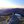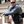0%
0%

# Inductance design tools

Python programs and library to make coil design easier.

Similar projects worth following
182 views
This project contains the math behind Serge Stroobandt's web page converted to a javascript library for calculation, and some additional functions to scan across coil parameters and print the values that would be shown from Serge's site.

Using a simple program loop and your initial design parameters, you can scan through different coil designs to maximize a parameter for your project.

For example, suppose you need a 25 uH coil with high Q. You can specify a wire diameter and coil value, then scan through all possible widths and lengths of coil: the program will interpolate the number of turns needed to achieve the inductance at the width and length, then print out the Q (and other information) for that coil. From this you can choose a design with a high calculated Q value.

You can do the same operation to optimize a coil for shortest wire, or smallest total volume, or whatever the designer needs.

The typical coil formula for inductance (the one you find on Wikipedia) is only accurate for audio frequencies, it tops out at about 100 KHz.

At RF frequencies, a handful of competing issues come into play, that are negligible at lower frequencies, to make your coil a different actual value. Most inductance measuring devices work at 10 KHz or 100 KHz and track the geometric formula shown above, so the engineer has no reason to believe the coil is not as designed.

For example, at higher frequencies the reactance from the inter-winding capacitance becomes non-negligible and subtracts from the inductive reactance. The capacitive reactance gets larger with higher frequencies, and at a high enough frequency the capacitive reactance equals the inductive reactance and you have a self-resonant single component (with no inductance).

Furthermore the geometric formula says nothing about the Q of a coil, so that designing an efficient coil with high Q is a complicated matter.

The complete formula for coil parameters is presented on Serge Stroobandt's web page and takes everything into consideration, including frequency and wire plating.

This project contains the math behind that web site converted to a javascript library for calculation, and some additional functions to scan across coil parameters and print the values that would be shown from Serge's site.

Using a simple program loop and your initial design parameters, you can scan through different coil designs to maximize a parameter for your project.

For example, suppose you need a 25 uH coil with high Q. You can specify a wire diameter and coil value, then scan through all possible widths and lengths of coil: the program will interpolate the number of turns needed to achieve the inductance at the width and length, then print out the Q (and other information) for that coil. From this you can choose a design with a high calculated Q value.

You can do the same operation to optimize a coil for shortest wire, or smallest total volume, or whatever the designer needs.

• 1
Github instructions

Instructions for using the programs are available on GitHub.

• 2
Use CoilCalc to characterize an existing coil
```#
# Example coil: Diameter 50mm, Length 200mm, with 35 turns
#
> CoilCalc --D=50 --l=200 --N=35 --d=1.44 --f=13.562

# QOIL™ — https://hamwaves.com/qoil/ — v20181217
#   Coil design 2023-03-11 12:20
# INPUT
#   mean diameter of the coil    D = 50.0 mm
#   number of turns              N = 35.0
#   length of the coil           ℓ = 200.0 mm
#   wire or tubing diameter      d = 1.44 mm
#   design frequency             f = 13.562 MHz
#   The (plating) material is annealed copper.
# INTERMEDIATE RESULTS
#   winding pitch                p = 5.71 mm
#   physical conductor length    ℓ_w_phys = 5501.4 mm
#   effective pitch angle        ψ = 2.09°
# RESULTS
#   Effective equivalent circuit
#     effective series inductance @ design frequency          L_eff_s = 16.652 μH
#     effective series reactance @ design frequency           X_eff_s = 1419.0 Ω
#     effective series AC resistance @ design frequency       R_eff_s = 1.438 Ω
#     effective unloaded quality factor @ design frequency    Q_eff   = 986
#   Lumped circuit equivalent
#     f-independent series inductance; geometrical formula    L_s     = 14.105 μH
#     series AC resistance @ design frequency                 R_s     = 1.032 Ω
#     parallel stray capacitance @ design frequency           C_p     = 1.5 pF
#   Self-resonant frequency                                   f_res   = 26.342 MHz
# DONATE
#   If this calculator proved any useful to you,
#   please, consider making a one-off donation
#   towards keeping me and the server up and running.
#   Thank you!
```
• 3
Use CoilScanL with an existing coil form
```#
# Make a 12uH coil using an existing 48mm form
#
> CoilScanL --LTarget=12 --DForm=48 --lMin=20 --lMax=250 --lInc=10 --d=1.44 --f=13.562

# CoilScan 2023-03-11 13:21
#
# LTarget = 12, d = 1.44, p = 6, f = 13.562
#
# DForm =  48, lMin  =  20, lMax = 250, lInc =  10
#
# D(mm),   l(mm), Q(plot),      Q,       N,    L(uH), wLen(m), Res(MHz), pitch(mm), Err, Cmd
49.44,   30.00,     621,     621   16.77,    12.00,     2.61,    34.32,      1.79,   0, "CoilCalc --D=49.440 --l=30.000 --N=16.770 --d=1.440 --f=13.562"
49.44,   40.00,     843,     843   17.89,    12.00,     2.78,    34.44,      2.24,   0, "CoilCalc --D=49.440 --l=40.000 --N=17.890 --d=1.440 --f=13.562"
49.44,   50.00,     947,     947   18.98,    12.00,     2.95,    34.40,      2.63,   0, "CoilCalc --D=49.440 --l=50.000 --N=18.980 --d=1.440 --f=13.562"
49.44,   60.00,     981,     981   20.03,    12.00,     3.11,    34.26,      2.99,   0, "CoilCalc --D=49.440 --l=60.000 --N=20.030 --d=1.440 --f=13.562"
49.44,   70.00,     986,     986   21.03,    12.00,     3.27,    34.05,      3.33,   0, "CoilCalc --D=49.440 --l=70.000 --N=21.030 --d=1.440 --f=13.562"
49.44,   80.00,     995,     995   21.98,    12.00,     3.41,    33.79,      3.64,   0, "CoilCalc --D=49.440 --l=80.000 --N=21.980 --d=1.440 --f=13.562"
49.44,   90.00,     983,     983   22.87,    12.00,     3.55,    33.52,      3.93,   0, "CoilCalc --D=49.440 --l=90.000 --N=22.870 --d=1.440 --f=13.562"
49.44,  100.00,     974,     974   23.72,    12.00,     3.69,    33.23,      4.22,   0, "CoilCalc --D=49.440 --l=100.000 --N=23.720 --d=1.440 --f=13.562"
49.44,  110.00,     972,     972   24.53,    12.00,     3.81,    32.94,      4.48,   0, "CoilCalc --D=49.440 --l=110.000 --N=24.530 --d=1.440 --f=13.562"
49.44,  120.00,     972,     972   25.31,    12.00,     3.93,    32.65,      4.74,   0, "CoilCalc --D=49.440 --l=120.000 --N=25.310 --d=1.440 --f=13.562"
49.44,  130.00,     957,     957   26.05,    12.00,     4.05,    32.37,      4.99,   0, "CoilCalc --D=49.440 --l=130.000 --N=26.050 --d=1.440 --f=13.562"
49.44,  140.00,     939,     939   26.76,    12.00,     4.16,    32.09,      5.23,   0, "CoilCalc --D=49.440 --l=140.000 --N=26.760 --d=1.440 --f=13.562"
49.44,  150.00,     923,     923   27.45,    12.00,     4.27,    31.82,      5.46,   0, "CoilCalc --D=49.440 --l=150.000 --N=27.450 --d=1.440 --f=13.562"
49.44,  160.00,     909,     909   28.11,    12.00,     4.37,    31.56,      5.69,   0, "CoilCalc --D=49.440 --l=160.000 --N=28.110 --d=1.440 --f=13.562"
49.44,  170.00,     897,     897   28.74,    12.00,     4.47,    31.30,      5.91,   0, "CoilCalc --D=49.440 --l=170.000 --N=28.740 --d=1.440 --f=13.562"
49.44,  180.00,     886,     886   29.35,    12.00,     4.56,    31.06,      6.13,   0, "CoilCalc --D=49.440 --l=180.000 --N=29.350 --d=1.440 --f=13.562"
49.44,  190.00,     876,     876   29.94,    12.00,     4.65,    30.82,      6.35,   0, "CoilCalc --D=49.440 --l=190.000 --N=29.940 --d=1.440 --f=13.562"

```

Share

## Similar Projects

Project Owner Contributor

### Nuclear Magnetic Resonance for EveryoneAndy Nicol

Project Owner ContributorTom Meehan